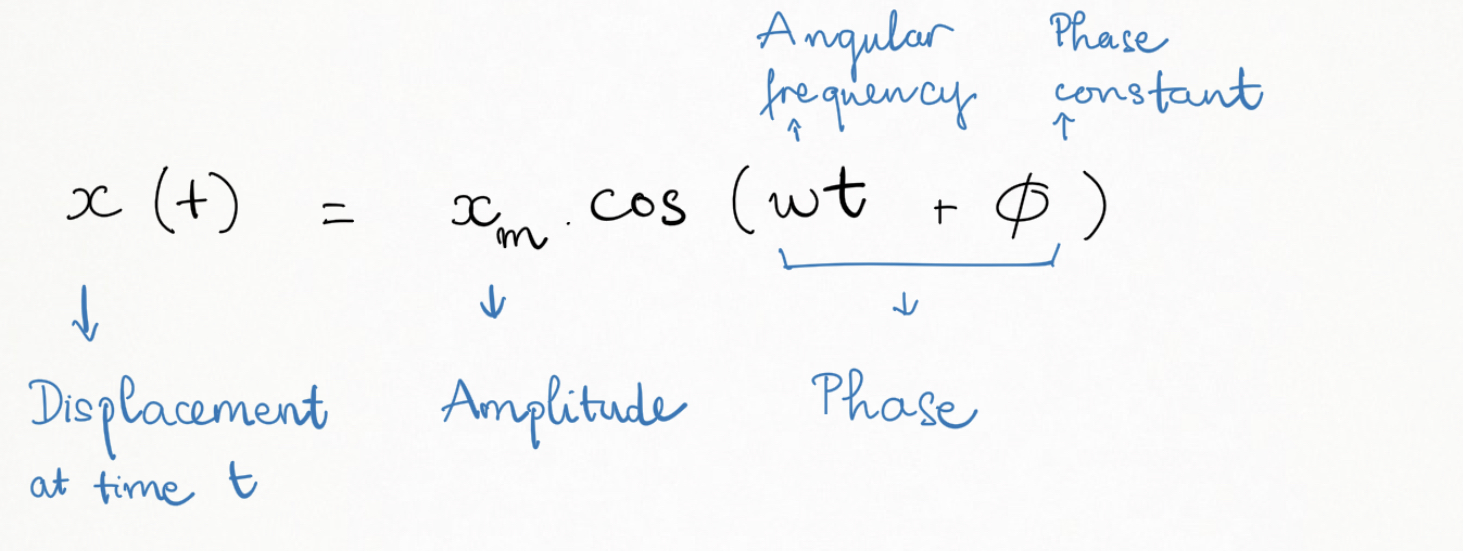Having trouble writing your personal statement?

MCAT Content / Periodic Motion / Amplitude Frequency Phase

### Amplitude, frequency, phase

Topic: Periodic Motion

Periodic motion or harmonic motion is any motion that repeats at regular intervals. Simple harmonic motion is a sinusoidal function of time t.

Periodic motion is observed in mass on a spring, simple pendulum, molecular vibration etc.The maximum displacement from equilibrium is known as the amplitude (always positive as only magnitude is considered).

One complete repetition of the motion is called a cycle. The duration of each cycle is the period. The frequency (f) is the number of cycles per unit time (t).

f=1/t.

For example, if a newborn baby’s heart beats at a frequency of 120 times a minute, its period (the interval between beats) is half a second as 60/120 = 0.5. Large frequencies means short periods.

Some motion is best characterized by the angular frequency (ω). The angular frequency refers to the angular displacement per unit time and is calculated from the frequency (f) with the equation:

ω=2πf.

The phase of the motion is the argument of the cosine function. Phase varies with time, so does the value of the cosine function and the displacement of the wave. φ is called the phase angle or phase constant, it defines the position of the particle when t=0.Practice Questions

The mechanics of standing balance

MCAT Official Prep (AAMC)

Physics Question Pack Passage 2 Question 7

Physics Question Pack Passage 2 Question 9

Physics Question Pack Passage 2 Question 10

Physics Question Pack Passage 2 Question 11

Physics Question Pack Passage 20 Question 113

Practice Exam 4 C/P Section Passage 3 Question 15

Key Points

• Periodic motion (harmonic motion) repeats at regular intervals.

• Periodic motion can be described by amplitude, frequency and phase in a sinusoidal function.

• Frequency can be calculated by doing 1/t which is the number of cycles in that period of time.

Key Terms

Amplitude: distance between the rest position and the crest of the wave. Proportional to intensity.

Period: the duration of one cycle in a repeating event

Frequency: frequency is the number of occurrences of a repeating event per unit of time.

Angular frequency (ω): ω=2πf.

Phase: of the wave is the argument of the cosine function

Periodic motion: any motion that repeats at regular intervals.

Billing Information